# IBPS Clerk Mains Quantitative Aptitude Questions 2019 – (Day-13)

Dear Aspirants, Our IBPS Guide team is providing new pattern Quantitative Aptitude Questions for IBPS Clerk Mains 2019 so the aspirants can practice it on a daily basis. These questions are framed by our skilled experts after understanding your needs thoroughly. Aspirants can practice these new series questions daily to familiarize with the exact exam pattern and make your preparation effective.

[WpProQuiz 7562]

Caselet

Directions (1 – 5): Read the following information carefully and answer the given questions.

In one of the college there are 1300 employees. The college has five departments – CSE, EEE, ECE, Civil and Mech. Out of the total number of female employees in the college, 30% work in CSE department, 22% work in ECE department, 18% work in EEE department, 12% work in Mech department and remaining 90 female employees work in Civil department. Out of the total number of male employees in the college, 18% work in CSE department, 20% work in ECE department, 30% work in Mech department, 22% work in EEE department and the remaining employees work in Civil department.

1) The total number of male employees working in the civil and EEE department together is approximately what percent of the total number of employees (both male and female) working in CSE and ECE department together?

a) 45%

b) 43%

c) 47%

d) 42%

e) 49%

2) If the male employees in ECE department is increase by 20%, the male employees in the Civil department increase by 10%, 25% of male employee increased by Mech department and 16 male employees Joins CSE department and the number of male employees in the EEE department is same, what is the percentage increase in the number of male employees in the college?

a) 12.5%

b) 14.5%

c) 16.5%

d) 10.5%

e) None of these

3) If 50 female employees from CSE department are transferred to the Mech department and 20 male employees from Mech department are transferred to the CSE department, what is the ratio of the number of female employee to the number of male employees in the Mech department after the transfer the employees?

a) 1: 2

b) 2: 5

c) 3: 2

d) 5: 3

e) None of these

4) What is the average number of employees (both male and female) who work in CSE, Mech and ECE department together?

a) 268

b) 258

c) 288

d) 298

e) None of these

5) What is the difference between the number of employees (both male and female) working in EEE department and the number of employees (both male and female) working in Civil department?

a) 76

b) 66

c) 86

d) 96

e) None of these

Number series

Directions (6 – 10): The questions below are based on the given Series-I. The series-I satisfy a certain pattern, follow the same pattern in Series-II and answer the questions given below.

6)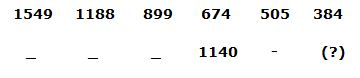a) 850

b) 900

c) 920

d) 825

e) 720

7)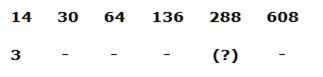a) 202

b) 196

c) 144

d) 112

e) 214

8)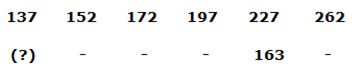a) 65

b) 73

c) 87

d) 53

e) 51

9)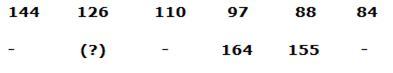a) 210

b) 187

c) 193

d) 171

e) 157

10)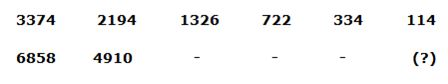a) 524

b) 612

c) 670

d) 718

e) 656

Directions (1 – 5):

18% of female employees work in Civil department = 90

Total number of female employees in the college = 500

Number of female employees in CSE department = 500 * 30/100 = 150

Number of female employees in ECE department = 500 * 22/100 = 110

Number of female employees in EEE department = 500 * 18/100 = 90

Number of female employees in Mech department = 500 * 12/100 = 60

Total number of male employees in the college = 1300 – 500 = 800

Number of male employees in CSE department = 800 * 18/100 = 144

Number of male employees in EEE department = 800 * 22/100 = 176

Number of male employees in ECE department = 800 * 20/100 = 160

Number of male employees in Mech department = 800 * 30/100 = 240

Number of male employees in Civil department = 800 * 10/100 = 80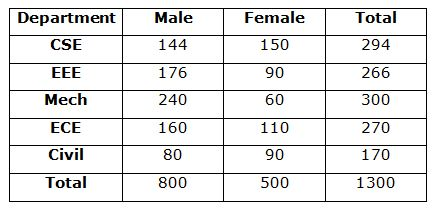Number of male employee in Civil department = 80

Number of male employee in EEE department = 176

Total male of Civil and EEE = 256

Total number of employees in CSE department = 294

Total number of employees in ECE department = 270

Total employees of ECE and CSE = 564

Required percentage = 256 * 100/564 = 45%

Number of male employees in ECE department = 160 * 120/100 = 192

Number of male employees in Civil department = 80 * 110/100 = 88

Number of male employees in Mech department = 240 * 125/100 = 300

Number of male employees in CSE department = 144 + 16 = 160

Number of male employees in EEE department = 176

New total number of male employees in the college = 916

Required percentage = (916 – 800) * 100/800 = 14.5%

After transferred the number of female employees in Mech department

= 60 + 50 = 110

After transferred number of male employees in Mech department

= 240 – 20 = 220

Required ratio = 110: 220 = 1: 2

Average = (294 + 300 + 270)/3 = 864/3 = 288

Difference = 266 – 170 = 96

Directions (6-10):

Series I pattern:

1549 – 192 (361) = 1188

1188 – 172 (289) = 899

899 – 152 (225) = 674

674 – 132 (169) = 505

505 – 112 (121) = 384

Series II pattern:

2015 – 192 (361) = 1654

1654 – 172 (289) = 1365

1365 – 152 (225) = 1140

1140 – 132 (169) = 971

971 – 112 (121) = 850

Series I pattern:

14 * 2 + 2 = 28+2 = 30

30 * 2 + 4 = 60 + 4 = 64

64 * 2 + 8 = 128 + 8 = 136

136 * 2 + 16 = 272 + 16 = 288

288 * 2 + 32 = 576 + 32 = 608

Series II pattern:

3 * 2 + 2 = 6 + 2 = 8

8 * 2 + 4 = 16 + 4 = 20

20 * 2 + 8 = 40 + 8 = 48

48 * 2 + 16 = 96 + 16 = 112

112 * 2 + 32 = 224 + 32 = 256

Series I pattern:

137 + 15 = 152

152 + 20 = 172

172 + 25 = 197

197 + 30 = 227

227 + 35 = 262

Series II pattern:

73 + 15 = 88

88 + 20 = 108

108 + 25 = 133

133 + 30 = 163

163 + 35 = 198

Series I pattern: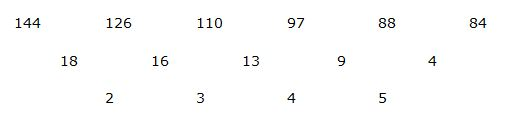Series II pattern: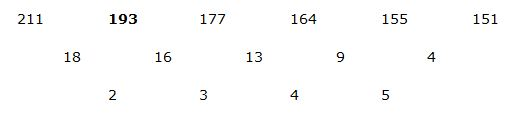Series I pattern:

153 – 1 = 3374

133 – 3 = 2194

113 – 5 = 1326

93 – 7 = 722

73 – 9 = 334

53 – 11 = 114

Series II pattern:

193 – 1 = 6858

173 – 3 = 4910

153 – 5 = 3370

133 – 7 = 2190

113 – 9 = 1322

93 – 11 = 718

5 1 vote
Rating